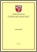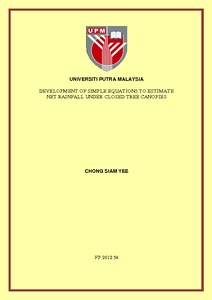Simple Search:

# Development of simple equations to estimate net rainfall under closed tree canopies

## Citation

Chong, Siam Yee (2012) Development of simple equations to estimate net rainfall under closed tree canopies. Masters thesis, Universiti Putra Malaysia.

## Abstract / Synopsis

Many interception models have been developed. These models, however, are complex, data-demanding, and often usable only for a specific vegetation type. The focus of this study was to develop and validate a two- and a three-coefficient equation for full canopies for oil palm, rubber, and pine trees. Throughfall and stemflow data from seven past studies were used to determine the best-fit coefficients for the two equations. The three-coefficient equation was Pn = Pg x exp [- (0.3443 – (Pg / (58.9748 + Pg)) x 0.1639)] and the two-coefficient equation was Pn = 0.7724 x Pg – 0.5845 (R2 = 0.91), where Pn and Pg are net and gross rainfall, respectively. To validate these two equations, field data were collected. Rain gauges were used for rainfall measurement and recorded in five minutes interval by data loggers. Ten rain gauges were used for throughfall measurement and arranged along the North-South direction with a 10-m interval. Three sampled trees were selected randomly for stemflow measurement and one rain gauge was installed at a nearby open area. Two error indexes were used as a goodness-of-fit measure, namely: index of agreement and normalized mean absolute error. Results showed that both the two and three-coefficient equations performed nearly equally well. The equations predicted net rainfall with errors between 12 to 23% (ranked as “Fair” to “Good” in terms of overall equation accuracy) and with an index of agreement of more than 90%. Results showed that these two equations can be used with fairly accurate estimations of throughfall and net rainfall, and, to a lesser degree, stemflow. The estimation errors were most probably because the canopy and rainfall characteristics were not taken into account in the two equations.Preview
PDF
FP 2012 54R.pdfView Item